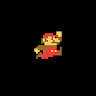C++ program to remove spaces from a string

Removing spaces from a string.

In this article we will learn how to code a C++ program to remove spaces from a string.  So we will be iterating the input string using while loop and then we will check if the character iterated is blank space or not. If the iterated character is not a blank space than we store it in the new string else we will not. In this way the spaces are removed from the string.Algorithm:

• Initialize the variables and accept the input.
• Initialize while loop and terminate it at the end of string.
• Iterate each character through the loop.
• Store each character except spaces in the new string.
• Print result.

CPP programming code to remove spaces from a string

#include <iostream>
using namespace std;
int main()
{

//Initializing variables.
char str, str_no_spc;
int i=0, j=0 ;

//Accepting inputs.
cout<<"Enter the string:\n";
gets(str);

//Iterating each character of string.
while(str[i] != '\0')
{
if(str[i] != ' ')
{

str_no_spc[j++] = str[i];
}

i++;
}
str_no_spc[j] = '\0';
//Printing result.
cout<<"The string after removing all the spaces is:\n"<<str_no_spc;

return 0;
}
Output:
Enter the string
PREP INSTA
The string after removing all the spaces is:
PREPINSTA

2 comments on “C++ program to remove spaces from a string”

•#include
#include
#include
using namespace std;
int main()
{
string s;
getline(cin,s);
int length=s.length();
int i=0;
while(s[i]!=’\0′)
{
if(s[i]==’ ‘)
{
for(int j=i;j<length;j++)
{
s[j]=s[j+1];
}
length–;
}
i++;
}
cout<<s;
return 0;
}0
•Asish

#python3
x=input()
a=[d for d in x]
b=[]
for i in range(len(a)):
if a[i]!=’ ‘:
b.append(a[i])
for j in range(len(b)):
print(b[j],end=”)1